CSAPP（一）
in 技术 with 0 comment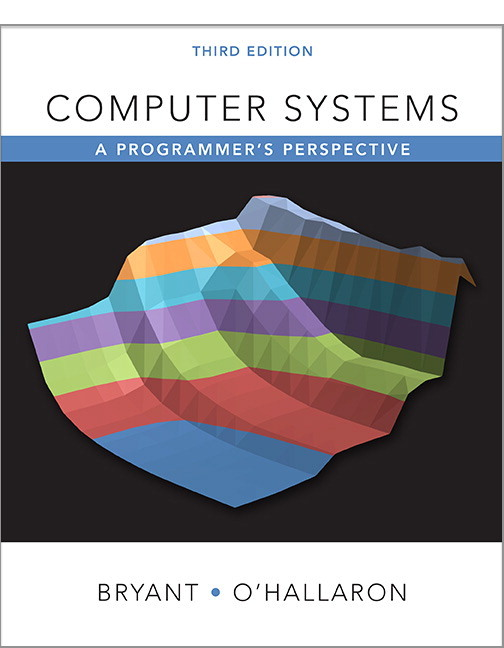# 第一章 计算机系统漫游

​ 一个总体观，计算机系统是由软件和硬件组成的，共同来运行应用程序，程序开始是ASCII文本，然后被编译器和链接器翻译成二进制可执行文件。

• c语言是贝尔实验室的Dennis Ritchie在1969～1973创建的（个人）。一本Kernighan和Ritchie的经典著作《K&R》
• 四个阶段的程序（预处理器、编译器、汇编器、链接器）构成了编译系统
• 硬件包括：总线、IO设备、主存、处理器、
• 文件是对IO设备的抽象，虚拟内存是对主存和磁盘IO设备的抽象，进程则是对处理器、主存和IO设备的抽象。

# 第二章 信息的表示和处理

## 2.1 信息存储

### 2.1.1 十六进制表示法### 2.1.2 字数据大小

• c语言数据类型的典型大小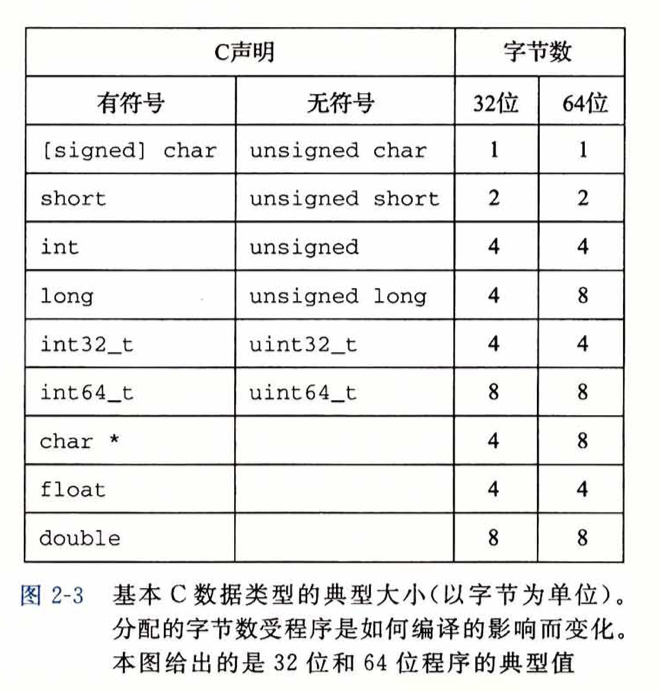• 其中int32_t和int64_t是固定为4个字节和8个字节。

### 2.1.3 寻址和字节顺序

• 大端法（big endian）
• 小端法（little endian）————最低有效字节在最前面（大多数Intel兼容机都只使用小端模式）
• Android和IOS都是只运行小端模式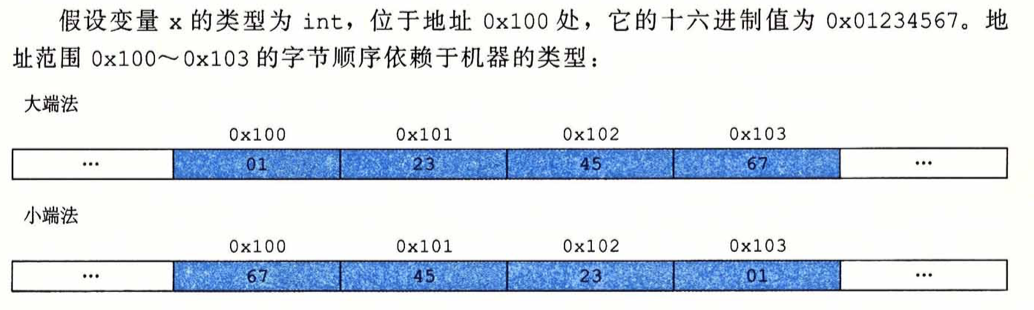### 2.1.6 布尔代数

• 布尔代数扩展到位向量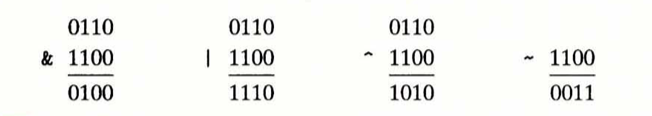### 2.1.7 C语言中的位级运算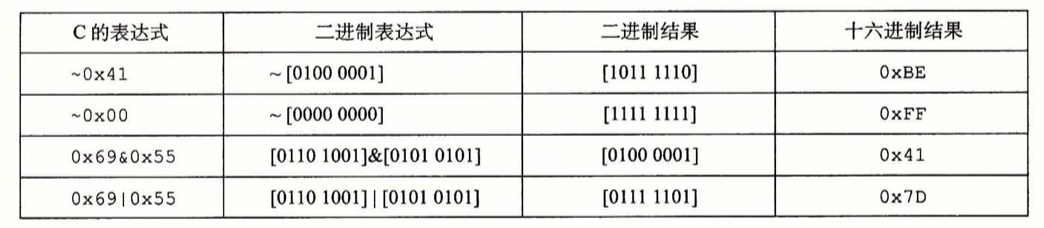### 2.1.8 C语言中的逻辑运算

• 逻辑运算中 非零的都是TRUE，只返回0或1，分别表示TRUE or FALSE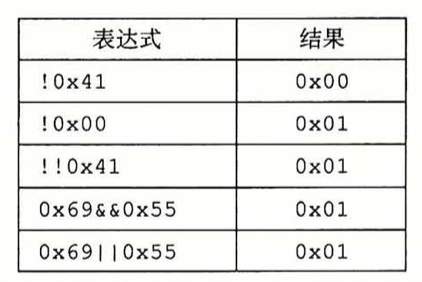• 其中!!0x41只有当后面的十六进为0x00才会返回0，否则都返回1，是lab中常用的技巧

### 2.1.9 C语言中的移位运算

• 算数右移：>>, 补的数字是最高有效位的值
• 逻辑右移：>>>，只补0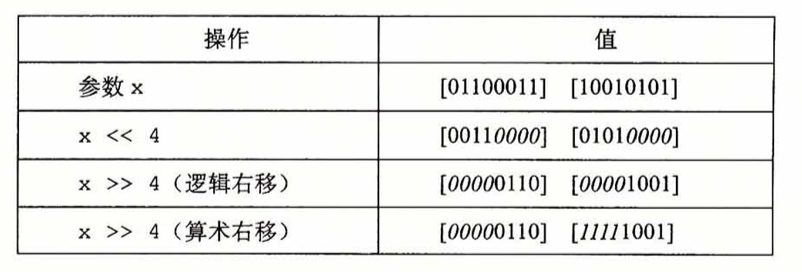• 左移：都是补0即可。
• 几乎所有的编译器/机器组合都对有符号数使用算术右移。且对于无符号数，右移都必须是逻辑的。

## 2.2 整数表示

### 2.2.1 整型数据类型

• 32位中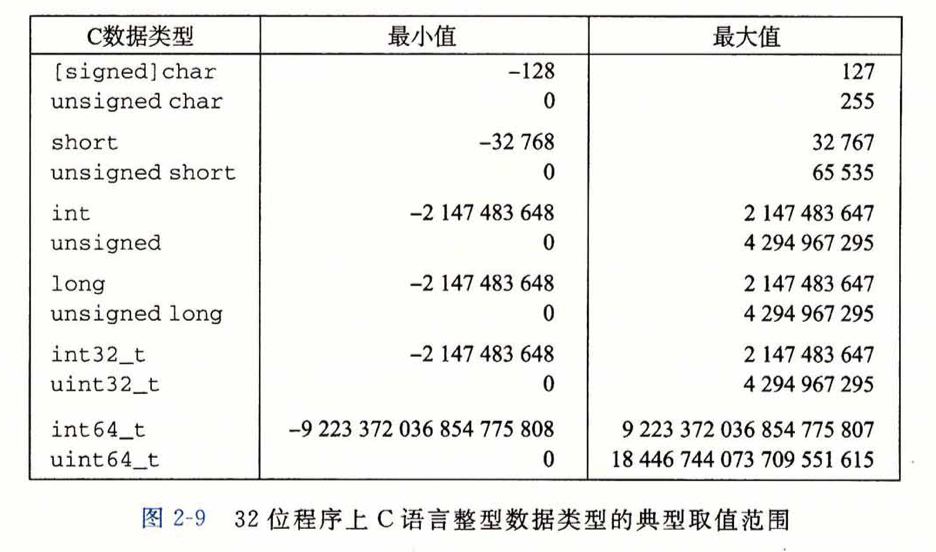• 64位中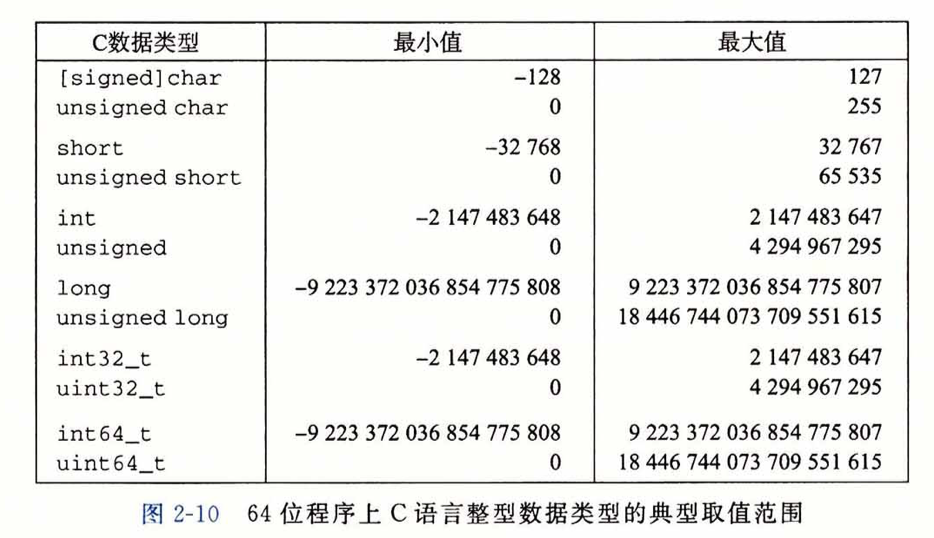• 有一个值得注意的特点是取值范围不是对称的————负数的范围比正数的大1

### 2.2.2 无符号数的编码（8421码）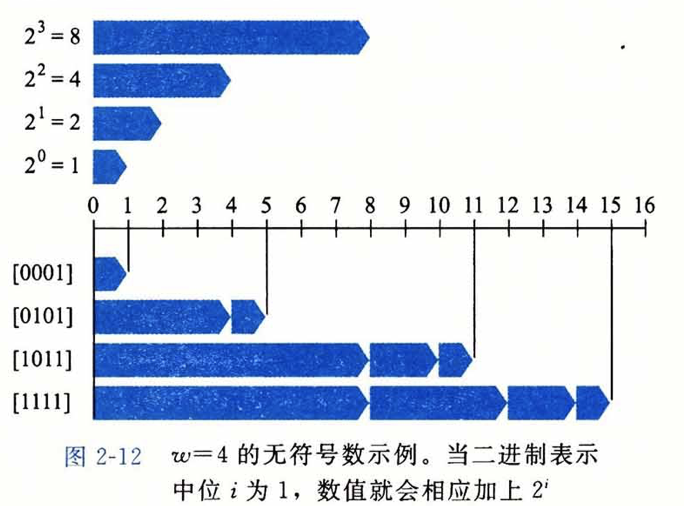### 2.2.3 补码编码[two’s-complement]（大多数机器都对整数使用补码编码）

• 将字的最高有效位解释为负权（negative weight），也称为符号为权重为-2^w-1^
• 符号位被设为1时表示负，0为非负。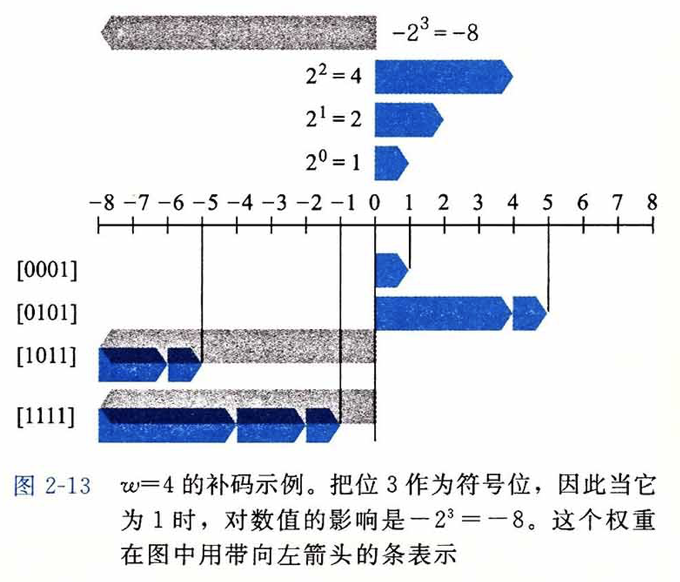• 一些重要的数字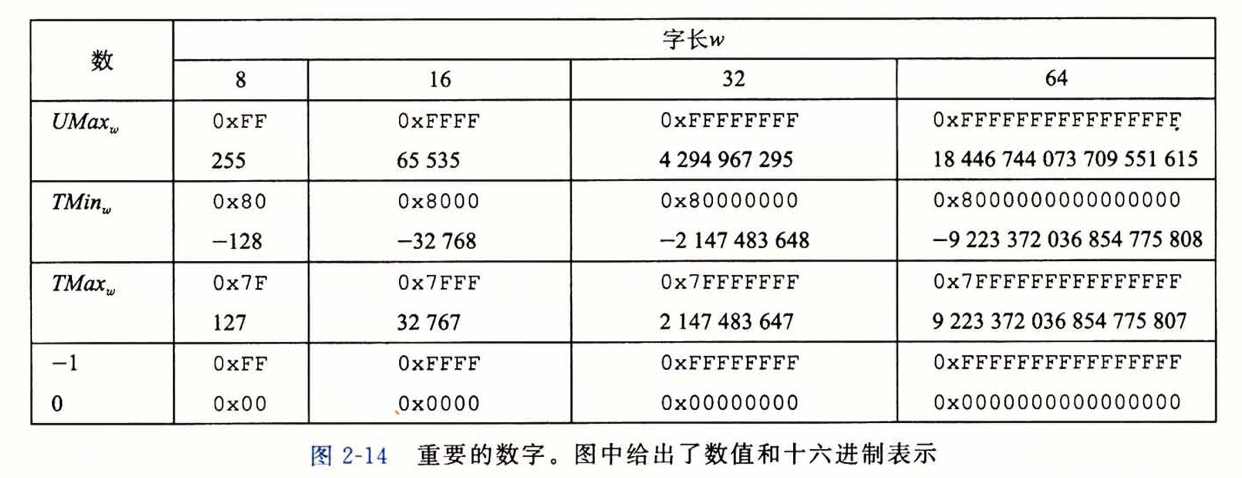• 补码的范围不对称 $|TMin| = |TMax| + 1$，即TMin没用对应的正数。

• $12345$的补码表示为，即0x3039
• $-12345$的补码表示为 , 即0xcfc7
• 取相反数的技巧，各位取反+1 -x == (~x+1)
• short类型下表示不了53191，unsigned short可以，位表示为 ,与补码中的$-12345$一样，可以看出有相同的位表示但是编码方式不同所表示的含义也会不同
• 同时 $12345+53191=65536=2^{16}$
• -1 和 UMax 有同样的位表示（都是全1的串）。数值0在两种表示方式中都是全0的串。
• 有符号数的另外两种表示方法：

• 反码(Ones‘ Complement)

除了最高位的权为 $-(2^{w-1}-1)$ 而不是$-2^{w-1}$之外，其他和补码是一样的

• 原码(Sign-Magnititude)

最高有效位是符号位，用来确定剩下的位是取正权还是负权

• 这两种表示方法都有一个奇怪的地方，对0有两种编码方式。把[00….0]都解释为+0，而-0在原码中表示为[10…0]，而在反码中表示为[11…1]。现在几乎没用机器用反码了。

### 2.2.4 有符号数和无符号数之间的转换

• 上面有提到$12345+53191=65536=2^{16}$，所以当一个有符号数映射为它相应的无符号数的时候，负数转换成了大的整数，而非负数不变。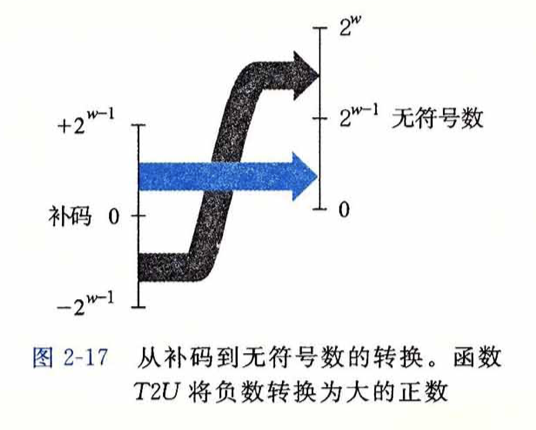• 无符号数转换为补码的时候 $0～2^{w-1}$不变，大于$2^{w-1}$的，则映射为负（即$u-2^w$）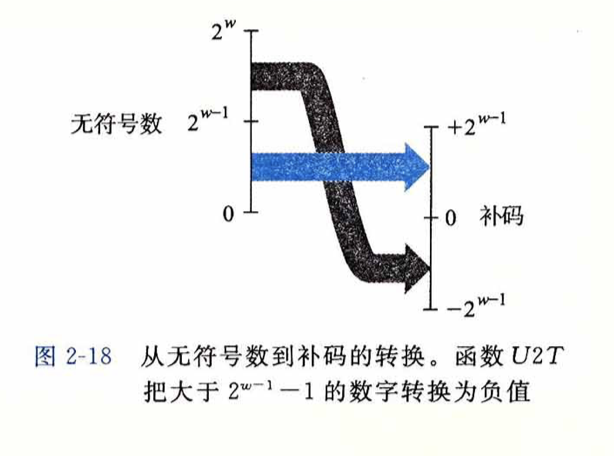### 2.2.5 C语言中的有符号数和无符号数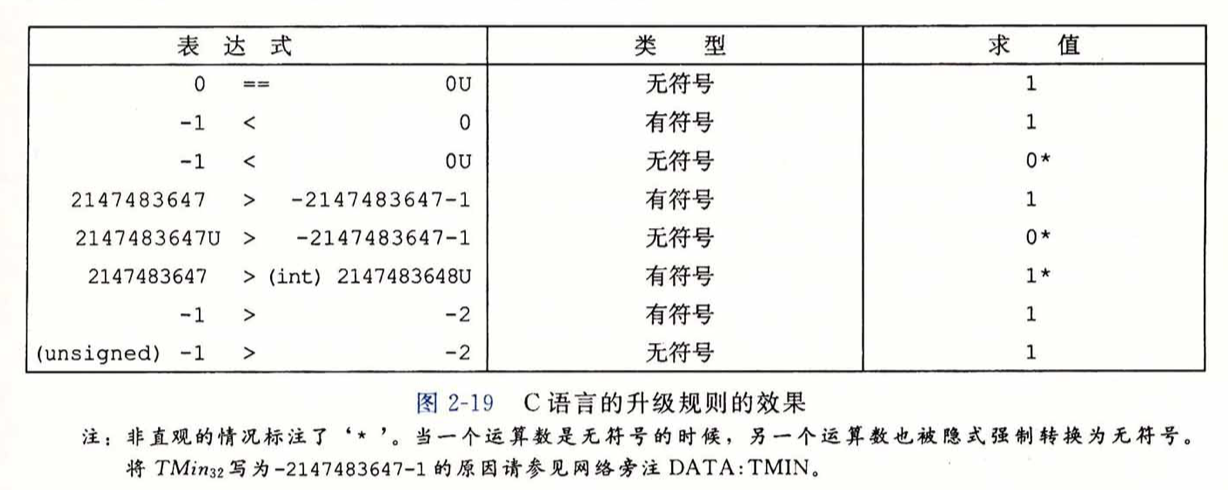### 2.2.6 扩展一个数字的位表示

• 无符号数的零扩展，开头加0即可
• 补码数的符号扩展

• 三位表示扩展到四位  和  都表示-3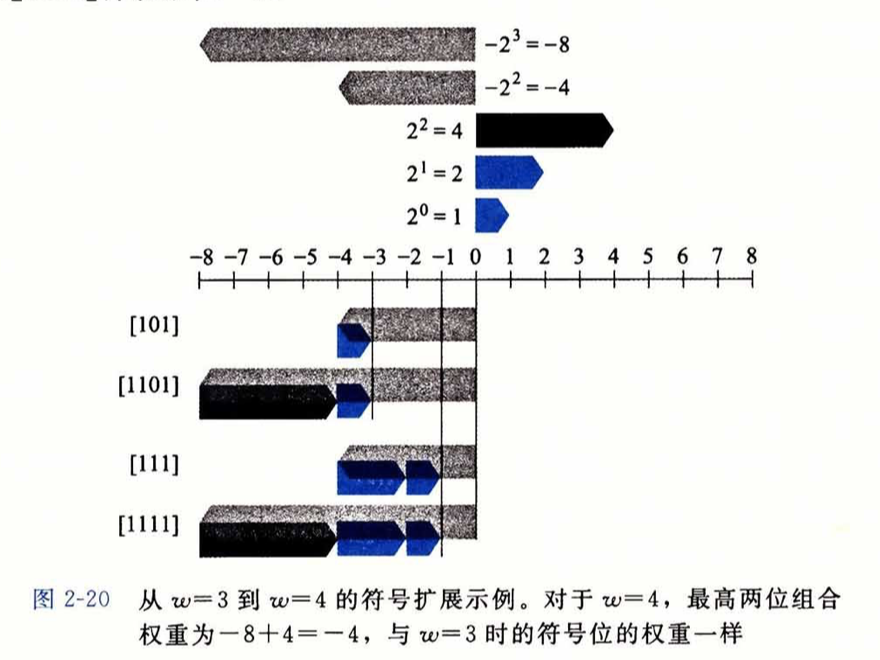### 2.2.7 截断数字

• 当减少表示一个数字的位数的时候可能会改变他的值—导致溢出。
• 53191 从32位截断到16位之后就变成了-12345

### 2.2.8 几个有符号数和无符号数之前隐式强制类型转换导致的漏洞

1.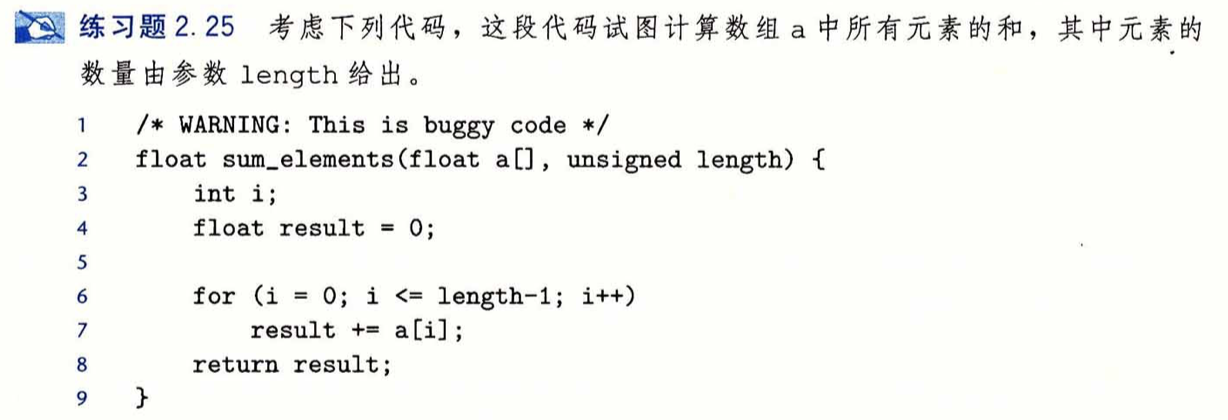代码第2行中 由于使用了unsigned类型，所以当length为0时，计算length-1 会得到UMax，会导致数组越界。改为int即可

1.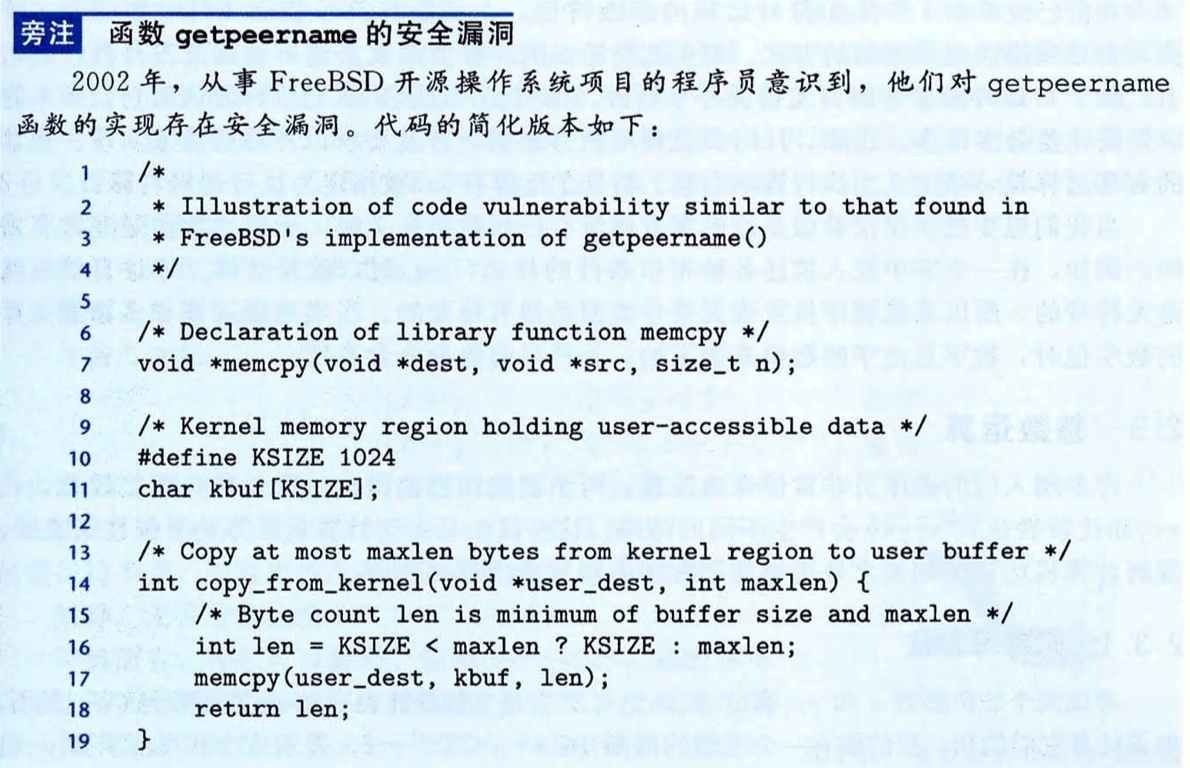代码7行的位置，size_t使用的是unsigned int(32) 及 unsigned int(64)，当它为负的时候，会变为一个非常大的正整数

## 2.3 整数运算

### 2.3.1 无符号加法

• 如果$x+y<2^w$ ，和的$w+1$位表示中的最高位为0，丢弃不会改变数值
• 如果$2^w<x + y<2^{w+1}$，和的$w+1$位表示中的最高位为1，丢弃它就相当于减去了$2^w$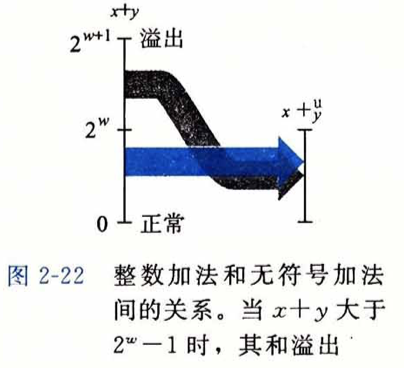### 2.3.2 补码加法

• 给定$x,y$的范围，即 $-2^{w-1}\leq x,y\leq2^{w-1}-1$，则其和的范围就是$-2^w\leq+y\leq 2^w -2$。如果想要精准表达可能需要w+1位
• 当$x+y\geq2^{w-1}$时，发生正溢出，截断的结果从和数中减去$2^w$
• 当$x+y<-2^{w-1}$ 时，发生负溢出，截断的结果是从和数中加上$2^w$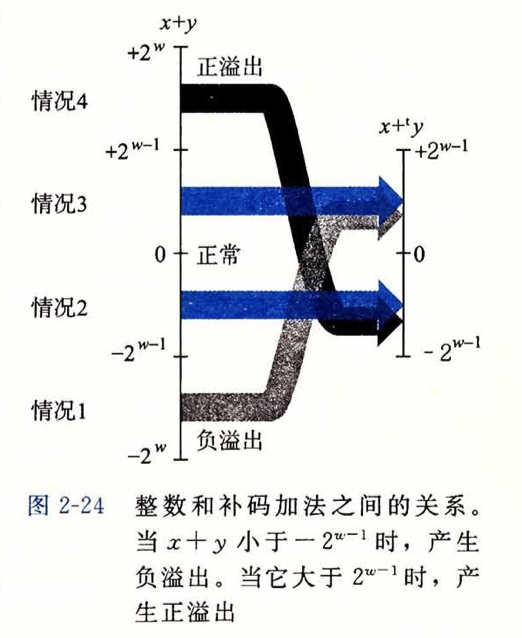### 2.3.3 补码的非

• $TMin$是自己的加法逆元，即$-TMin=TMin$，对于其他任何的数x都有-x作为其加法逆元，使得$-x+x=0$
• $TMin+TMin = -2^{w-1} +(-2^{w-1} ) = -2^w$，会导致负溢，由上节中所说可知，负溢出的时候需要和数加上$2^w$，所以导致结果为零，$TMin$的加法逆元为自己。
• 补码非的另一种位级表示就是，每一位求补再加1，即$-x$和$～x+1$的结果完全一样（后面一个是波浪号）

### 2.3.4 无符号乘法

• 范围是$0\leq x,y\leq2^w-1$的无符号数相乘的范围是$0$到$(2^w-1) ^2$，需要$2w$位来表示，但是产生的结果只有$w$位，则用低w位来表示结果，相当于模$2^w$

### 2.3.5 补码乘法

• 同样地，对于$-2^{w-1}\leq x,y \leq 2^w -1$的两个数相乘也需要$2w$位来表示，截断为低$w$位，相当于先模$2^w$ ,再把无符号数转换为补码。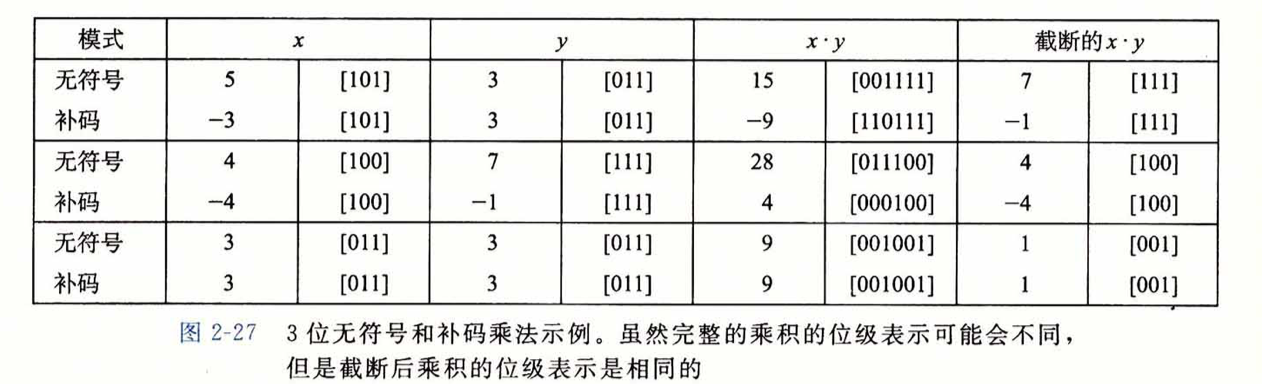### 2.3.6 乘以常数

• 很多机器上执行乘法都是很慢，但是其他整数运算比较快（加减法，位级运算和移位）。所以会尝试使用移位和加法运算的组合来代替乘以常数因子的乘法。
• 左移一个数相当于执行了一个与2的幂相乘的无符号乘法。
• 对于补码的乘法，补码的位级运算与其无符号运算等价
• $x * 14 = (x<<3) + (x << 2) + (x<<1)$

### 2.3.7 除以2的幂

• 大多数机器上除法要比乘法更慢。
• 除以2的幂也可以用移位运算来实现，无符号使用逻辑右移（补0），补码使用算术右移（补0）
• 除以2的无符号数除法，x>>k 产生数值$\lfloor x/2^k \rfloor$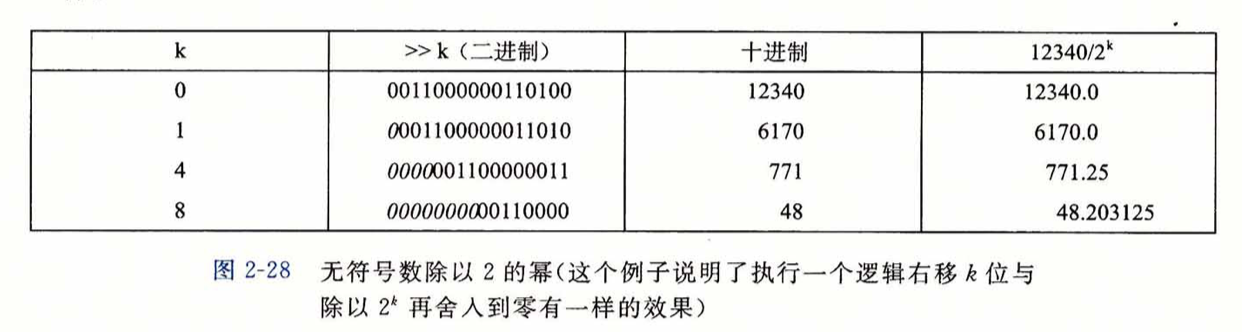• 对于补码的除法，向下舍入，x>>k 产生数值$\lfloor x/2^k \rfloor$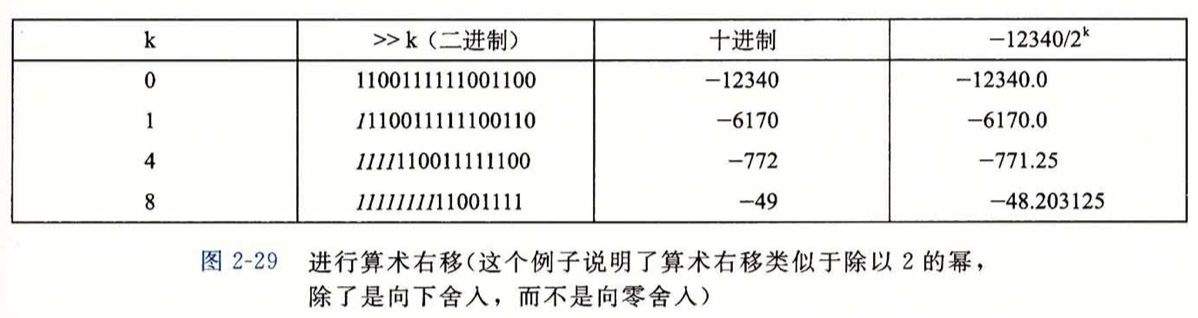• 对于补码的除法，向下舍入，$(x+(1<<k)-1)>>k$ 产生数值$\lceil x/2^k \rceil$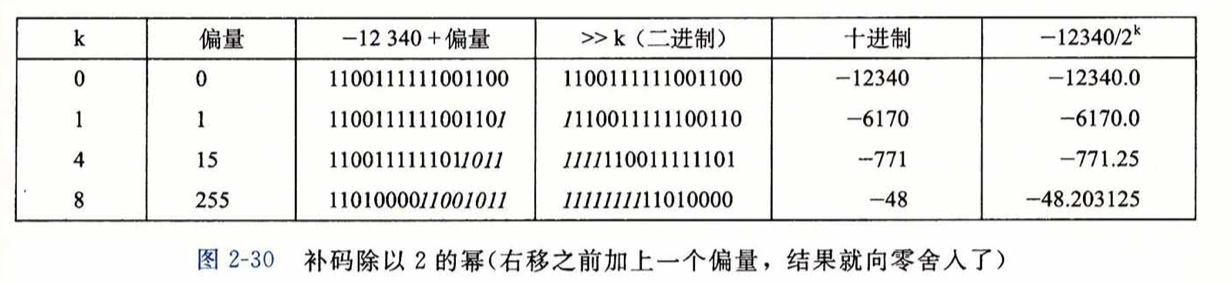## 2.4 浮点数

### 2.4.1 二进制小数

• 小数点右边是2的负幂，小数点左边是2的正幂
• 小数的二进制表示只能表示能够被写为$x\times2^y$的数，其他的数只能被近似的表示。

### 2.4.2 IEEE浮点表示（:star:）

• 用 $V = (-1)^s \times M \times 2^E$来表示一个数

符号（sign）s表示数的正负

尾数（significand）M是一个二进制小数，范围是 $1～2-\varepsilon$

阶码（exponent）E的作用是对浮点加权

• 使位表示划分为三个字段，一个单独的符号位s，k位阶码字段exp用来编码E，n位小数字段frac用来编码M。

在单精度float中，s=1位，k=8位，n=23位。得到32位的表示

在双精度double中，s=1位，k=11位，n=52位，得到64位的表示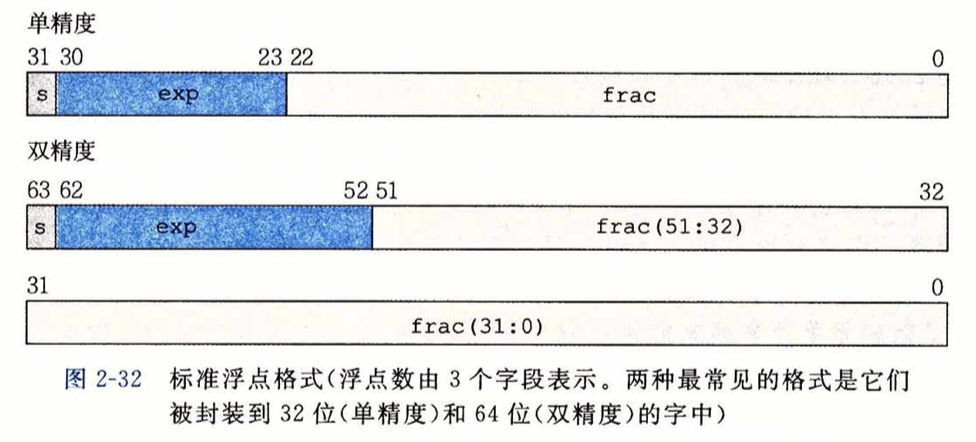• 单精度浮点数的分类，根据exp的不同来划分1. 如果exp不为全1或全0。规格化的值，阶码的值是 $E=e-127(单精度)$，尾数M=1+f（隐含的1开头），即表示f的时候是一个0到1的小数值，但实际上表示的是一个1到2之间的数字。
2. 当exp全部都为0。非规格化的值，阶码$E = 1 - 127$，尾数的值是$M=f$，无隐含的开头1。非规格化数有两个用途，一个是表示数值0的方法，因为使用规格化数的时候，有隐含的开头1，尾数M表示的值总是大于1的。另一个用途就是表示那些非常接近0的数。

• $+0.0$ 就是所有位都是0。
• $-0.0$ 是符号位s为1，其他所有位都是0。
3. 当exp全为1时，分两种情况：

• 小数域f全为0时，表示无穷大，且$s=0$表示$+∞$，$s\neq$0表示$-∞$
• 小数域f全为1时，表示NaN（Not a Number）

### 2.4.3 数字示例

• 假定的八位浮点格式示例。k=4的阶码位，n=3的小数位。$bias=2^{4-1} - 1 = 7$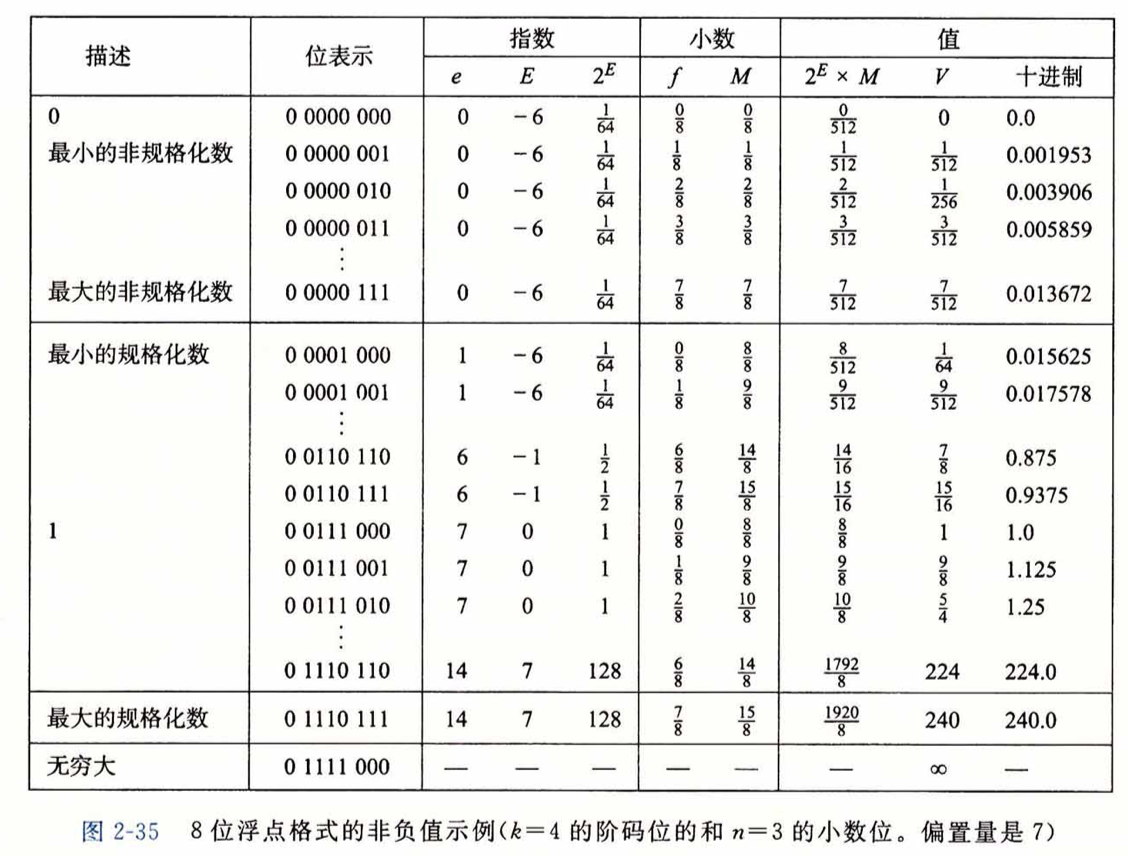• 在非规格化数中 定义$E=1-Bias$是为了平滑转变，比如上图中的最大非规格化数$\frac{7}{512}$和最小规格化数$\frac{8}{512}$
• 12345的浮点数表示法

• 二进制0x3039 [0011 0000 0011 1001]， 小数点左移13位得到$12345 = 1.1000000111001 _2 \times 2^{13}$
• 丢弃开头的1，并在末位加10个0构造出小数字段得到

[1000 0001 1100 1000 0000 000]

• 构造阶码字段，13加上偏置量127得140，二进制为，再加上符号位0
• 最终得到[0 <u>1000 1100</u> <u>1000 0001 1100 1000 0000 000</u>] 0x4640E400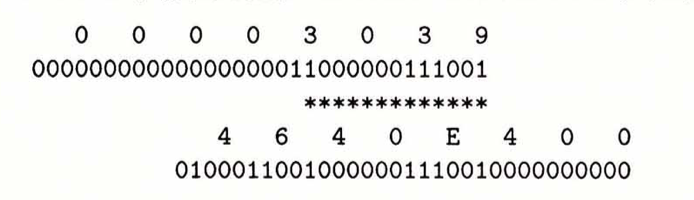### 2.4.4 舍入

• 向偶数舍入法：将最低有效位的值0认为是偶数，值1认为是奇数。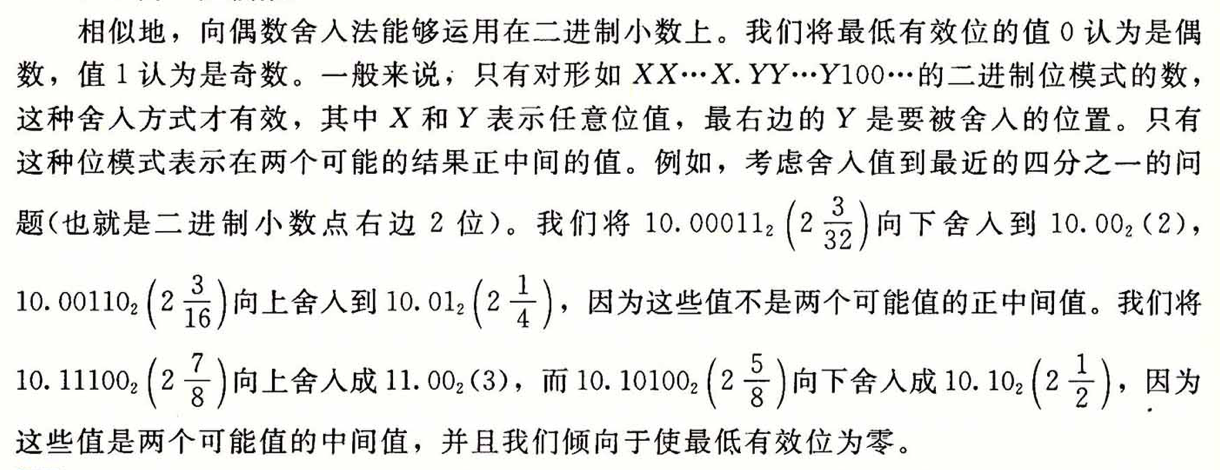### 2.4.5 浮点运算

• 浮点加法不具有结合性。(1e20 1e20) 1e-20 求值为+∞，1e20 (1e20 1e-20)为1e20
• 同样的浮点乘法也不具有结合性。1e20(1e20-1e20)为0.0 ,而1e20 1e20 - 1e20 * 1e20会得到NaN。